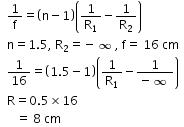Courses

# Test: Refraction By Lens

## 10 Questions MCQ Test Physics For JEE | Test: Refraction By Lens

Description
This mock test of Test: Refraction By Lens for JEE helps you for every JEE entrance exam. This contains 10 Multiple Choice Questions for JEE Test: Refraction By Lens (mcq) to study with solutions a complete question bank. The solved questions answers in this Test: Refraction By Lens quiz give you a good mix of easy questions and tough questions. JEE students definitely take this Test: Refraction By Lens exercise for a better result in the exam. You can find other Test: Refraction By Lens extra questions, long questions & short questions for JEE on EduRev as well by searching above.
QUESTION: 1

### We wish to make a plano convex lens of focal length 16 cm from glass having refractive index 1.5. It is to be used in air. What should be the radius of curvature of the curved surface?​

Solution:QUESTION: 2

### A convergent lens made of crown glass (refractive index 1.5) has focal length 20cm in air. If it is immersed in a liquid of refractive index 1.60, its focal length will be:​

Solution:

When the lens is in air, we have (from lens maker's equation), 1/20 = [(1.5/1) - 1) (1/R1- 1/R2).
We are not bothered about the signs of R1 and R2 since they are unknown quantities.
Even though we know that the convergent lens will become divergent in the denser medium, we write the lens maker's equation without bothering about the sign of its unknown focal length in the liquid. If if is the focal length in the liquid, we can write,
1/f = ((1.5/1.6) - 1) (1/R1 - 1/R2).
Dividing the first equation by the second, we obtain f/20 = 0.5x1.6/(-0.1) from which f = -160 cm

QUESTION: 3

### If R1 and R2 are the radii of curvature of a double convex lens, which of the following will have the largest power?​

Solution:

Using the lens maker’s formula we get
p= (μ−1) (1/R1​+1/R2​)
As we can clearly see, when R1= R2= 5cm then the maximum power is achieved.

QUESTION: 4

A concave lens of glass, refractive index 1.5, has both surfaces of same radius of curvature R. On immersion in a medium of refractive index 1.75, it will behave as a:​

Solution:

Consider refraction of light from infinity at first surface,
(μ2/v)​​−( μ1/u​)​=(μ2​−μ1/R)​​
(1.5​/ v1​)-(1.75/−∞)​=1.5−1.75​/−R
Consider refraction of this image from second surface,
​(1.75​/ v2)−(​1.5/v1)​=(1.75−1.5​)/R
Hence, v2​=3.5R
This is the image of light coming from infinity, therefore the focal length.
It is converging since focal length is positive.

QUESTION: 5

If two thin lenses of focal length f1 and f2 are held in contact then the focal length of the combination will be

Solution:
QUESTION: 6

A convex lens produces a real image m times the size of the object. What is the distance of the object from the lens?

Solution:

M=−ϑ /u ​=−m/1​
ϑ=−mu
(1/ϑ​)−(1/u)​=1/f
1/mu​+1/u​=−1/f​
(1+m)​/xu=−1/f​
u= (1+m)​f/(m)

QUESTION: 7

What is the unit of power of lens?

Solution:
QUESTION: 8

A double convex thin lens made of glass of refractive index 1.6 has radii of curvature 15 cm each. The focal length of this lens when immersed in a fluid of refractive index 1.63 is​

Solution:

1/f= ((μ2- μ1)/ μ1){(1/R1) –(1/R2)}
Refractive index of glass, μ2=1.6, refractive index of liquid, μ1=1.63
R1=15 cm ,R2=-15 cm
The focal length of this lens when immersed in a fluid is:
1/f=((1.6-1.63)/1.63){(1/15)-(1/-15)}
F=-416.67cm

QUESTION: 9

1 dioptre =​

Solution:

A dioptre (British spelling) or dioptre (American spelling) is a unit of measurement of the optical power of a lens or curved mirror, which is equal to the reciprocal of the focal length measured in metres. (1 dioptre = 1 m−1.) It is thus a unit of reciprocal length.

QUESTION: 10

If two thin lenses of power p1 and p2 are held in contact then the power of the combination will be​

Solution: